# How to get the opposite of an ABS value in Excel?

ABS in Excel stands for "Absolute Value". This function takes only one argument, as a cell reference or number directly, and then determines the required absolute value. This article guides learners about the process to generate the opposite of an ABS value in Excel by using the formula, and VBA code method. The formula method contains two basic formulas, to convert the value directly to the opposite value. While the VBA code method is a code-based method, that uses the VBA code to solve the provided task.

## Example 1: To get the opposite of an ABS value in excel by using the formula

### Step 1

Open the Excel sheet and type the provided data into the sheet. To understand the working properly, consider the below-given sample data.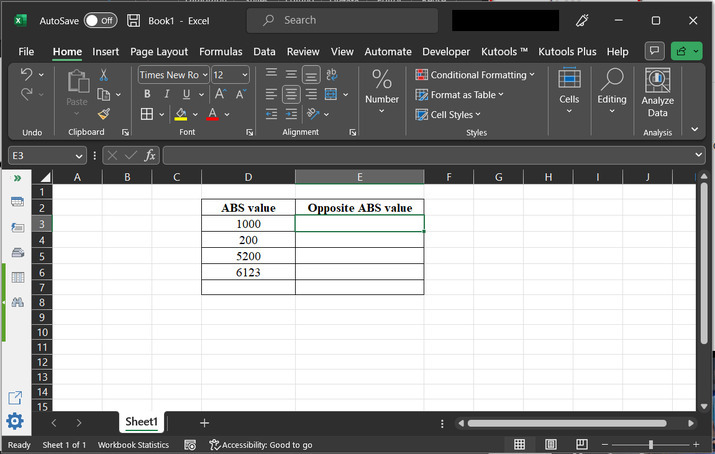### Step 2

After that go to the “E3” cell, and type the formula “=-(D3)” or “=D3*-1”. Both the formulas will perform the same task and will simply add a negative sign to the D3 cell value.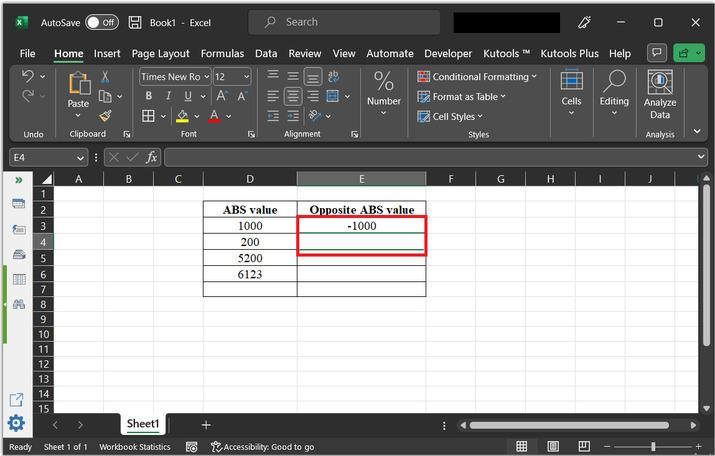### Step 3

Drag the results down to copy this same formula to the next available cells.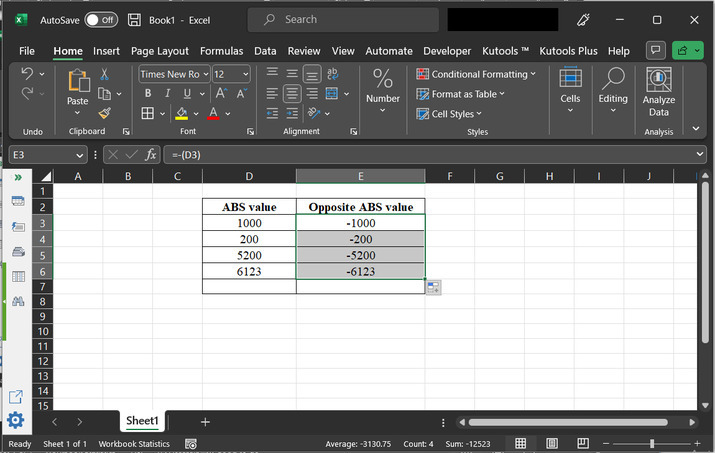## Example 2: To get the opposite of an ABS value in excel by using the VBA code

### Step 1

Consider the same worksheet. This time user can use the VBA code to generate the same result. Firstly, go to the Developer tab and click on “Visual Basic” under the code section. Consider the below provide image for a better understanding.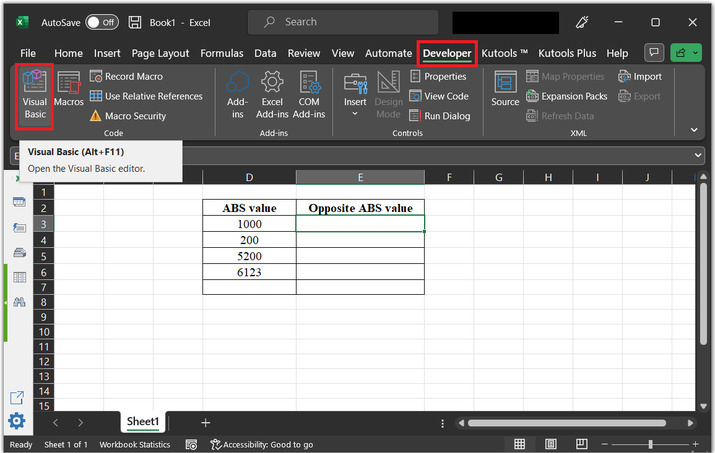### Step 2

This will open the “Microsoft Visual Basic for Applications” dialog box. As depicted below −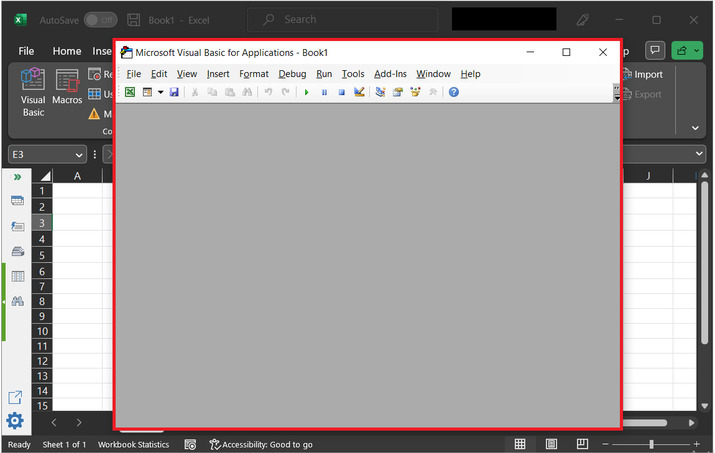### Step 3

In the opened dialog box, click on the “Insert” tab and then select the option “Module”. As shown below −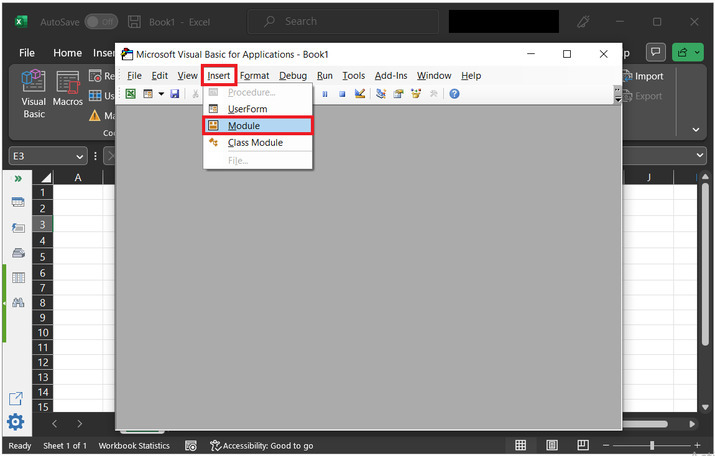### Step 4

This will open the code area. Here, user can type the required Visual basic code.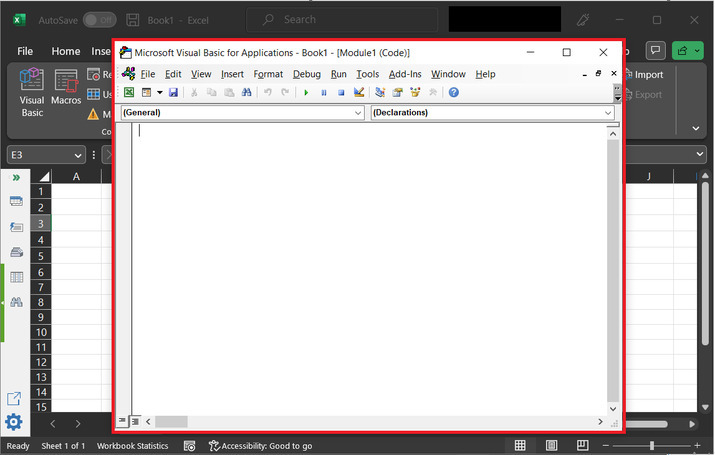### Step 5

Copy the below given code, to the code area −

' define function to convert positive abs value to negative
Public Function ConvertToNegative(ByVal num As Double) As Double
' if entered value is less than 0
If num >= 0 Then
' calculate negative number
ConvertToNegative = num * -1
' otherwise
Else
' display number as it is
ConvertToNegative = num
' end of if block
End If
' end of function
End Function


Please note that VBA code will work only if the code is entered properly by using the proper required syntax.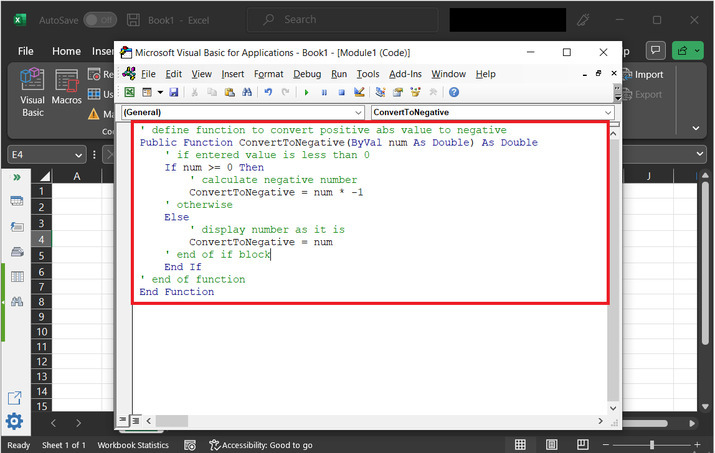### Step 6

Save the above code by using the key combination “Ctrl + S”. Again, go to the Excel sheet, and type the below-given formula −

“=ConvertToNegative(D3)”


The above-provided formula is nothing but a call made to the user-defined method.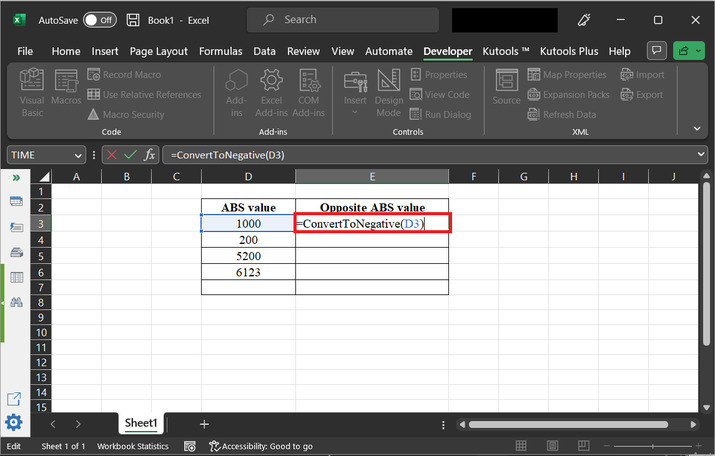### Step 7

The above function call will simply add a “-” in the front of D column values.### Step 8

Drag the lower corner of cell. This will help user to copy the result to all the required rows.## Conclusion

This article briefs learners about the practice to obtain the opposite ABS value. Two examples are given in this article. The first example is based on using the formula, and the VBA code is used in the second example. The proper syntax and code need to be written to run the defined function in the VBA module properly to get the opposite of an ABS value in MS Excel.

Updated on: 08-May-2023

106 Views